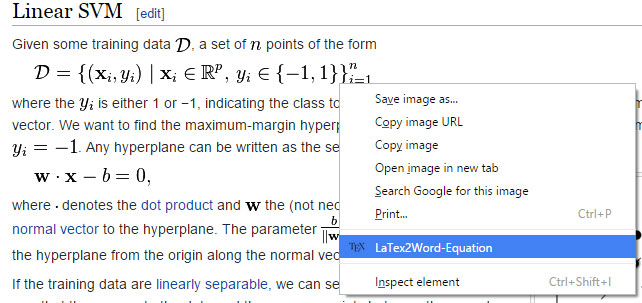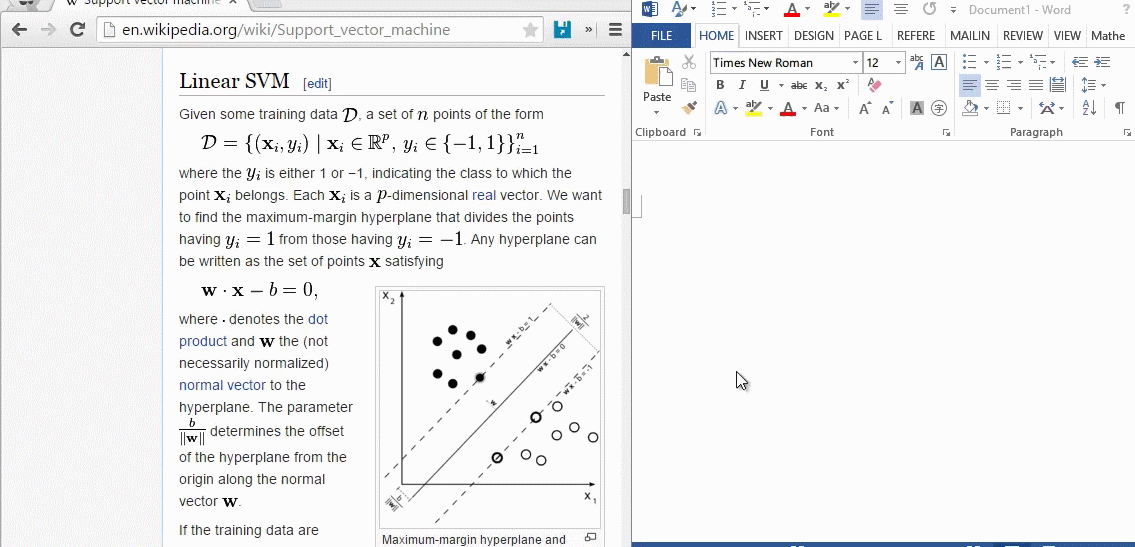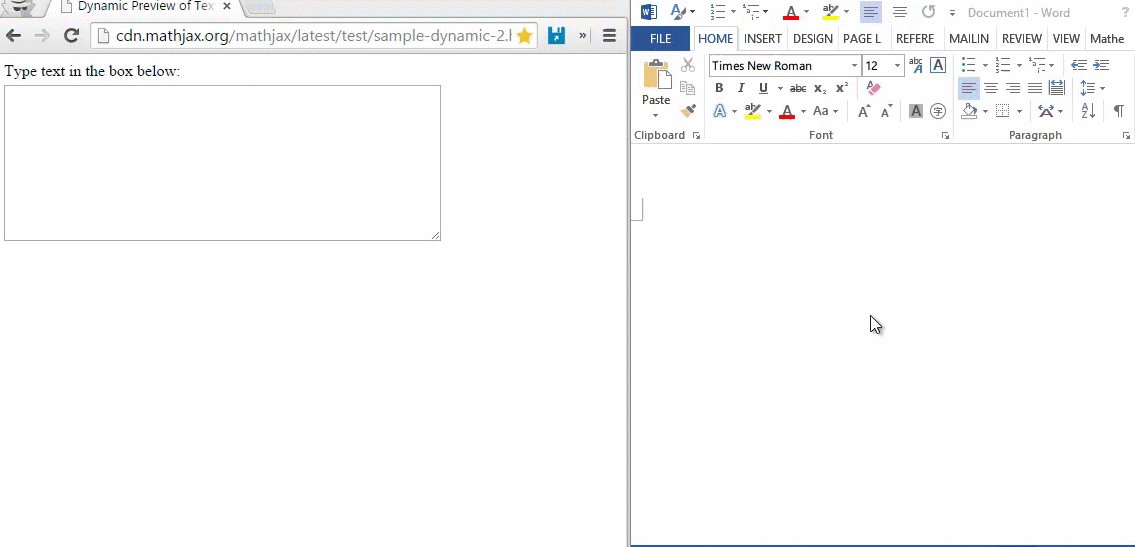# LaTeX2Word-Equation

Copy LaTeX Equations as Word Equations
Chrome Extension in the StoreRight click:# Demo

## Copy equations from Wikipedia## Copy equations from raw LaTeX math code

copy raw LaTex math code \alpha \beta \gamma to Word as the equation# References

Get A Weekly Email With Trending Projects For These Topics
No Spam. Unsubscribe easily at any time.
Javascript (1,158,774
Latex (10,318
Math (10,281
Chrome Extension (10,240
Mathjax (784